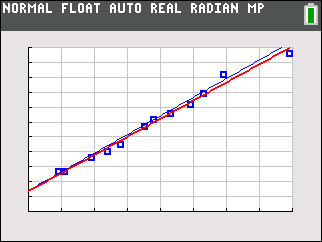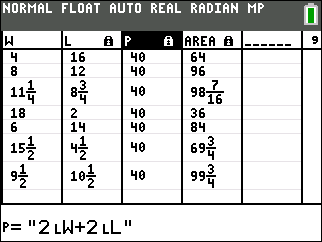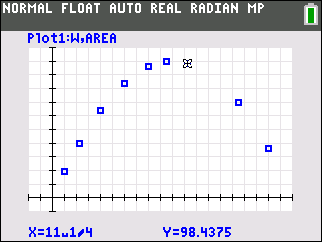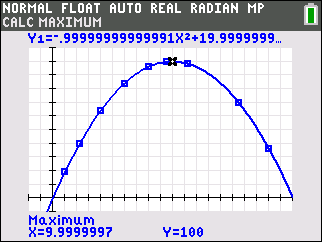••• ##### Device
• TI-84 Plus
• TI-84 Plus Silver Edition
•TI-84 Plus C Silver Edition
•TI-84 Plus CE

# Geometry: Old McDonald's Pigpen

by Texas Instruments#### Overview

In this activity, students explore – numerically, graphically, algebraically, and verbally – the mathematics involved in maximizing the area of a rectangle with a fixed perimeter.

#### Key Steps

•Students will graph scatter plots, analyze quadratic functions, and determine the maximum value of a parabola.

•Students will solve design problems that satisfy physical constraints by using geometric and algebraic methods.

•Students will observe numeric patterns, reason abstractly, and make connections between geometric figures and algebraic expressions.